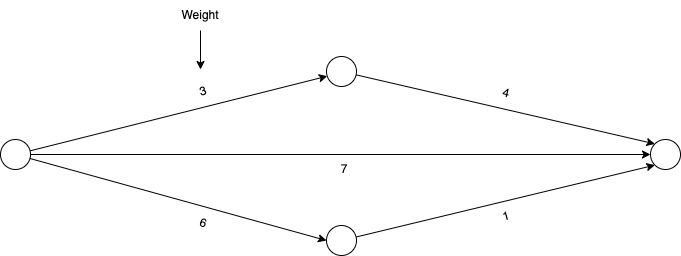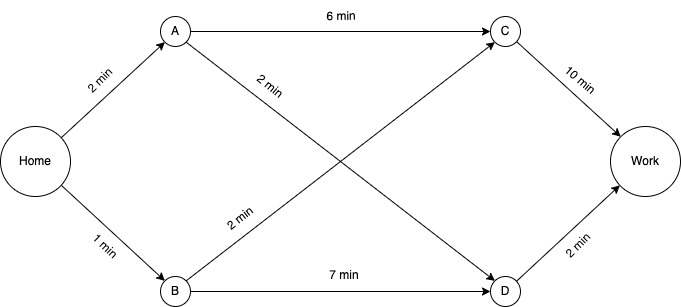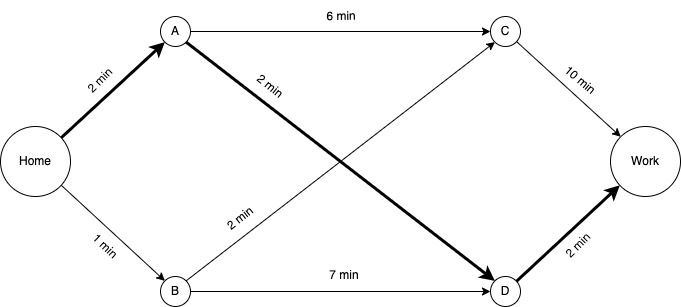# <BackWeighted graphs

In a previous article, we got acquainted with the general definition of a graph, its implementation in the form of an adjacency matrix, and the basic algorithm for breadth-first search. This algorithm is good, but it won't work for a weighted graph.

Important: In this article I will expand the functionality of the adjacency matrix from the last article. If you are not familiar with the code yet, you can do it here or read the previous article.

We will get acquainted with weighted graphs, Dijkstra's algorithm, and its implementation in the Rust programming language.

## What is a weighted graph?

A weighted graph is a graph that has a number associated with each edge, called a weight.

Here's what it looks like:Dijkstra's algorithm is used to find the optimal path in such graphs.

## Dijkstra's algorithm

Mark overslept, and he's late for work. He needs help choosing a quick path to work. This is what the graph of this problem looks like:The most optimal path looks like this:The main purpose of Dijkstra's algorithm is to find the path with the lowest cost.

The algorithm consists of several steps:

1. Find the node with the lowest cost.
2. Update the cost of the neighbors of this node.
3. Repeat until all nodes are passed.
4. Calculate the final path.

Let's apply the algorithm to our problem.

Step 1:

The first smallest neighbor B = 1 min;

The cost of passing the path Home -> B -> C = 3 min, and Home -> B -> D = 8 min.

Step 2:

The next node is A = 2 min;

The cost of passing the path Home -> A -> D = 4 min, and Home -> A -> C = 8 min.

After these steps we get the following data:

Home -> B -> C = 3 min - the most optimal way to point C, let's remember it

Home -> B -> D = 8 min

Home -> A -> D = 4 min - the most optimal way to point D, let's remember it

House -> A -> C = 7 min

After these steps, there are two nodes C and D.

Step 4:

The node with the lowest cost C = 3 min;

The cost of passing the way Home -> B -> C -> Work = 13 min.

Step 5:

The next node is D = 4 min;

The cost of passing the way Home -> A -> D -> Work = 6 min.

Gets that the fastest way to work is Home -> A -> D.

IMPORTANT: The edges of a weighted graph can be with negative weights. Dijkstra's algorithm does not work for such graphs, but there is a Bellman-Ford algorithm that will help solve the problem.

And now let's move on to the implementation of our algorithm.

## Implementation

Let's add a function for the Matrix structure that will calculate the index of the node with the minimum weight.

```use std::i32::MAX;
﻿
impl Matrix {
pub fn min_distance(&self, dists: &mut Vec<i32>, done: &mut Vec<bool>) -> usize {
let mut min = MAX;
let mut min_node = 0;
for v in 0..self.n {
if !done[v] && dists[v] <= min {
min = dists[v];
min_node = v;
}
}
return min_node;
}
}
```

Now we describe a basic version of the algorithm that calculates the optimal cost for each node of the graph. In `dist` we will store the optimal cost, where the index is the node number. In the `done` vector, we store information about passed nodes.

```impl Matrix {
pub fn dijsktra(&self, start_node: usize) {
let mut dists: Vec<i32> = vec![MAX; self.n];
let mut done: Vec<bool> = vec![false; self.n];
dists[start_node] = 0;
for _ in 0..(self.n - 1) {
let min_node = self.min_distance(&mut dists, &mut done);
done[min_node] = true;
let not_zero = self[min_node][adj_node] != 0;
let new_dist = dists[min_node] + self[min_node][adj_node];
&& not_zero
&& dists[min_node] != MAX
{
}
}
}
println!("Distances: {:?}", dists);
}
}
```

I want the algorithm to output the optimal path and cost for a particular node. Let's upgrade the algorithm. Let's create a path vector, the index of which corresponds to the node, and the index value preceding the optimal node. Even at the input of the algorithm, we will take the index of the node we are interested in.

```pub fn dijsktra(&self, start_node: usize, target_node: usize) -> Option<(i32, Vec<usize>)> {
let mut dists: Vec<i32> = vec![MAX; self.n];
let mut done: Vec<bool> = vec![false; self.n];
dists[start_node] = 0;
let mut path: Vec<usize> = vec![0; self.n];
for _ in 0..(self.n - 1) {
let min_node = self.min_distance(&mut dists, &mut done);
done[min_node] = true;
let not_zero = self[min_node][adj_node] != 0;
let new_dist = dists[min_node] + self[min_node][adj_node];
&& not_zero
&& dists[min_node] != MAX
{
}
}
}
if dists[target_node] == MAX {
return None;
}
let mut target_path = path[target_node];
let mut final_path = vec![target_node];
while !final_path.contains(&0) {
final_path.push(target_path);
target_path = path[target_path];
}
final_path.reverse();
return Some((dists[target_node], final_path));
}
}
```

Now is the time to test the algorithm on our graph.

```fn main() {

// Node 0 - Home

// Node 1 - A

// Node 2 - B

// Node 3 - C
﻿
// Node 4 - D

// Node 5 - Work
﻿
let mut graph: Matrix = Matrix::new(6, 0);
let res = graph.dijsktra(0, 5);
if res.is_some() {
let res = res.unwrap();
println!("Weights sum: {:?}", res.0);
println!("Path: {:?}", res.1);
}
}

Output:
Weights sum: 6
Path: [0, 1, 4, 5]
```

Hashtags:
#algorithms
#graphs
#rust
Share:

## Lates

### Composition of the IT development team

In this article we will look at the composition of the IT solution development team

#developmentprocess

In this article, we will begin our acquaintance with graphs, get acquainted with the breadth-first search algorithm (BFS) and implement the graph in the Rust programming language.

### What is the difference between outsourcing development and outstaffing an IT employee for development?

In this article we will understand what outsourcing and outstaff development are.

#developmentprocess

### UI/UX design: The creation process

In this article we will talk about the main steps in the process of creating UI/UX design.

#developmentprocess

### UI/UX design: Introduction

In this article, we begin to get acquainted with UI / UX design. This is the most important stage in the development of any visual application interface.

#developmentprocess

### Agile, Six Sigma and No Principle

In the last article, we started diving into the development process. The first stage of this process is planning. At this stage, the project manager, together with other team members, forms a pool of tasks in accordance with some kind of project management methodology.

#developmentprocess

### Meet the Pentest

We are beginning to consider one of the main methods of assessing the security of computer systems and networks for potential vulnerabilities - penetration testing

#pentest

### Reducing the implementation period of MVP

Let's figure out the timing of the implementation of the MVP.

#developmentprocess

#wannacode

### Testing an MVP concept

We will figure out how not to waste the budget on MVP development in vain

#developmentprocess

### Application Architecture Design: Introduction

In this article, we will talk about the process of creating the architecture of an IT solution.

#developmentprocess

### The terms of references: Structure

In this publication we will consider the universal structure of ToR

#developmentprocess

### Incorrect estimation of the cost of IT contractor services

Today we will talk about the incorrect assessment of the cost of developing IT solutions. This pain is one of the main ones for enterprises and startups, including IT contractors themselves.

#consultin

### Introduction to Design Patterns in Software Development

In this article, we will begin to dive into the world of optimizing application architecture using design patterns.

#designpatterns

### Choosing the direction of development for programming training

In this article, you will find out what areas of IT development there are, how they differ and in which they pay more

#wannacode

### OSI Model Levels

In this article, we will take a closer look at each of the levels of the OSI model

### Main types of application architecture

In this publication, we will look at what application architectures are

#developmentprocess

### 10 ways to use Rust Cargo

In this short article I have collected 10 ways to use the build system and package manager of the Rust programming language

### Documenting code in the Rust programming language

In this article, we will look at how documentation takes place in Rust and consider a very useful opportunity - writing tests through documentation.

#rust

### Introduction to the OSI model

In this article we begin to consider the fundamental model of network interaction - OSI

#networks

### CSS animation ripple

A simple example of how to implement ripple animation using HTML and CSS

#css

### What is the purpose of an ER-diagram in the development process?

Let's discuss in general terms what an ER diagram is and what it is used for.

#developmentprocess

### From concept to MVP

In this article, you will learn, by example, how to move from a concept to an MVP without unnecessary complications in the functionality of the product

#developmentprocess

### What are UML diagrams used for?

In this article we will talk about what UML diagrams are, what they are and where they are used

#developmentprocess

### Introduction to writing the terms of references

The Terms of Reference are an important part of the development process. In this article, we will begin to dive into this issue.

#developmentprocess

### Introduction to software development

Today, most companies are faced with IT development and often do not get what they want. In this article, we begin to dive into the process of creating IT solutions.

#developmentprocess

### From idea to concept

In this publication, we will talk about how the idea differs from the concept. Let's do this with an example of a specific goal

#developmentprocess

### Development Process: Planning

In this publication, we will begin to consider the development process. Let's start with the planning process.

#developmentprocess